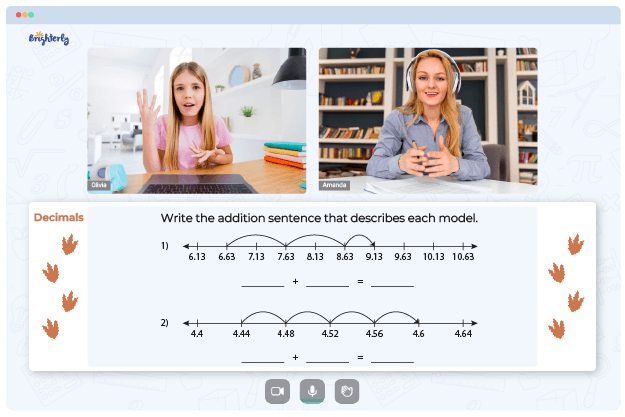# Comparing and Ordering Decimals Worksheets

Comparing and ordering decimals involves determining the relative values of decimal numbers and arranging them in ascending or descending order. Comparing and ordering decimals worksheets help students understand decimals.

Math for Kids

Is Your Child Struggling With Math?
1:1 Online Math Tutoring## Benefits of Using Ordering Decimals and Fractions Worksheets

Here are some of the benefits of using ordering decimals worksheets:

• Worksheets on ordering decimals help students better understand decimals and the decimal system.
• Comparing and ordering decimals worksheets with answer keys offer a focused and organized approach for students to improve their skills.
• Comparing ordering decimals worksheets can be used to set assessments.

Download free comparing and ordering decimals worksheets on Brighterly or you can get comparing and ordering decimals free worksheets on numerous math websites on the internet.

Need help with Decimals?• Does your child struggle with understanding the concept of decimals?
• Try learning with an online tutor.

Is your child finding it hard to understand decimals lessons? An online tutor could be of help.